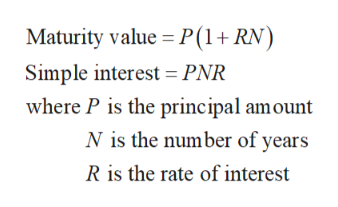# Find the maturity value and the amount of simple interest earned. Round to the nearest cent.2) \$5482 at 4.6% for 9 months

Question
15 viewshelp_outlineImage TranscriptioncloseFind the maturity value and the amount of simple interest earned. Round to the nearest cent. 2) \$5482 at 4.6% for 9 months fullscreen
check_circle

Step 1

Recall the follow...help_outlineImage TranscriptioncloseMaturity value P(1+RN Simple interest = PNR where P is the principal amount N is the number of years R is the rate of interest fullscreen

### Want to see the full answer?

See Solution

#### Want to see this answer and more?

Solutions are written by subject experts who are available 24/7. Questions are typically answered within 1 hour.*

See Solution
*Response times may vary by subject and question.
Tagged in

### Math